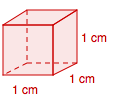### Home > MC1 > Chapter 7 > Lesson 7.1.1 > Problem7-6

7-6.

Imagine a cube that is $1$ cm wide, $1$ cm hight, and $1$ cm long.

1. Sketch the cube on your paper.2. What is the volume of this cube?

What is the area of the base? Use this information to help you find the volume.
Remember volume is in cubic units.

3. What is the surface area of this cube?

Remember that the surface area is the combined area of all of the faces.
What is the area of one of the faces? How many equal sized faces does a cube have?